## 3.2 The density distribution

The initial nebular model to be run was the simplest possible density distribution, a homogeneous spherical geometry, to reproduce the CELs. The chemical abundances were taken to be homogeneous. A first attempt was made by using a homogeneous density distribution of 2550 cm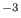with different inner and outer radii, deduced from the [OII], [SII] and [ClIII] lines (García-Rojas et al., 2009). However, the uniform density distribution did not match the ionization and thermal structures, so we examined different density distributions adopted based on radiation-hydrodynamics simulations (see e.g. Schönberner et al., 2005a; Schönberner et al., 2005b; Perinotto et al., 2004) to make the best fit to the observed CELs, and also to constrain the shell thickness. Some radiation-hydrodynamics results depict a radial density profile having the form, where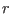is the radial distance from the center,the radial density dependence,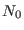the characteristic density,the outer radius. We finally adopted a density distribution with a powerlaw radial profile. Figure 4 illustrates the 3-D spherical density model constructed based on the radial density profile, and the ionizing source is located in the corner. We chose the characteristic density of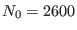cmand the radial density dependence of. The outer radius of the sphere is equal to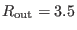arcsec and the thickness is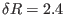arcsec. We examined distances, with values within the range of distances 2.2 and 5.8 kpc (Phillips, 2004, and references therein). The distance ofkpc found here, was chosen, because of the best predicted H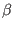luminosity, and it is within the range of distances 4.2 and 5.15 kpc estimated by Todt et al. (2010). Taking the angular diameter of 7 arcsec, we derive an outer radios ofcm at the given distance ofkpc.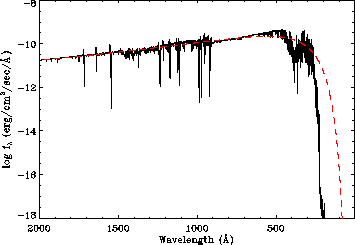Parameter Value Filling factor 0.056 Mass fraction 6.3 percent Number of knots 33 Knot size (arcsec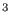)(cm) 3300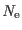(cm) 4012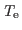(K) 4286

A second attempt (MC2) was to reproduce the observed ORLs by introducing a fraction of metal-rich knots into the density distribution used by the first model. The abundance ratios of He, N, and O relative to H in the metal-rich component are higher than those in the normal component. Two different bi-abundance models suggested by Liu et al. (2000): one assumes that H-deficient component with a very high density (cm) and a moderate temperature (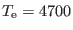K), which has been adopted in the bi-abundance photoionization model of Abell 30 (Ercolano et al., 2003b) and NGC 6153 (Yuan et al., 2011). However, H-deficient component in the second model introduced by Liu et al. (2000) has an 8 times lower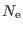and a 20 times lower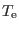than the normal component. After exploring both the assumptions, we adopted a dense metal-rich component with the H number density of 3300cm, which is roughly 1.7 times higher than the mean density of the surrounding material.

In Figure4, the 3-D spatial distribution of metal-rich knots (cells) are shown as a darker shade inside the density model of normal abundances. The gas-filling factor in the density model MC1 was kept at unity, while the inclusion of 33 metal-rich cells in the normal abundances nebula in the model MC2 (see Table 4) leads to gas-filling factors of 0.056 for the metal-rich component. It means that the dense metal-rich inclusions occupy 5.6 percent of the total gaseous volume of the nebula. Higher values of the gas-filling factor for the metal-rich component require lower values of the number density in order to reproduce the ORLs, but they may not provide the best match. We note that the filling factor parameter in MOCASSIN is still set to 1.0, which can have a major role in emission-line calculations. For example, reducing the MOCASSIN filling factor parameter from 1.0 to 0.5 decreases the nebular Hluminosity by a factor of 2 and increases theOIII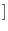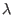5007/Hflux ratio by 9 percent, so the nebula cannot be reproduced.For our bi-abundance model, we assume 33 metal-rich knots are uniformly distributed inside the diffuse warm nebula. However, Ercolano et al. (2003b) adopted a dense core surrounded by a less dense outer region in the bi-abundance model of Abell 30. Moreover, the metal-rich inclusions were distributed in the inner region of the diffuse warm nebula in the bi-abundance model of NGC 6153 (Yuan et al., 2011). Adopting the current knot density (3300cm) in PB8, different distributions of metal-rich knots require totally different N/H and O/H abundance ratios and filling factors, which may not be in pressure equilibrium with their diffuse warm nebula. We note that ADFs of Abell 30 and NGC 6153 are very large, and are not similar to moderate ADFs of PB8. Moreover, the effective temperatures of the central stars of Abell 30 and NGC 6153 are 130000 and 90000K, respectively, which are hotter than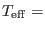52000K in PB8. This indicates that the central star of PB8 is in an early stage of its evolutionary path towards becoming a white dwarf, and should be younger than the hot central stars of Abell 30 and NGC 6153.

The physical size of the metal-rich knots also play an important role in making an ionization structure to reproduce the N II and O II ORLs. It is found that the physical size of (0.008)parsec(atkpc) can well produce the observed ORLs. Reducing the physical size will lead to an increase in the number of knots, which also need different density or/and N/H and O/H abundance ratios to match the observation. Smaller sizes will contribute to higher ionization and more thermal effects (see also Yuan et al., 2011), so we may not be able to reproduce electron temperatures estimated from the ORLs (see Section4.2). A larger physical size will also decreases the number of knots, but it needs different density or/and different elemental abundances of N and O, which may not make metal-rich inclusions in pressure equilibrium with the surrounding gas.

Ashkbiz Danehkar
2018-03-28